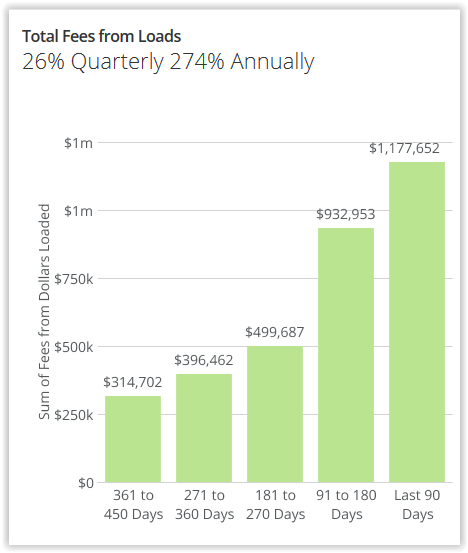# Can I have 2 numbers (% and absolute value) in the summary number section of a card?

I am looking for a way to have 2 numbers in the summary number section (% and absolute value). How can I display both?

Thanks,

Celine

• Hi @CelineH,

There is a way to do that through a Beastmode. Basically you will need to create a formula concatenating the folowwing things:

CONCAT(

(FormulaForThePercentage),

'% sign plus the name you give your calculation',

(FormulaForTheAbsoluteValue),

ones you create your beastmode make sure to check the box "Apply to Summary Number" and save.

https://dojo.domo.com/t5/Domo-Dojo-Contest-January-2016/quot-Best-Beast-Mode-Formula-quot/idi-p/7840

This is an example , a formula I currently use. hope it helps you

CONCAT(FLOOR(
(SUM(CASE
/
SUM(CASE
,'% Quarterly ',
FLOOR(
(SUM(CASE
/
SUM(CASE
,'% Annually')• Add a ROUND(XXXX , 4) to the equation. CONCAT( ROUND((SUM(`Calculation Use 1`)/COUNT(`Phone`)),4)*100,'% Conversion Rate',' ',',', COUNT(`Phone`),' ','Total Leads')

• This works great and we have employed situations like this as well. One item to keep in mind, is if you use this, you cannot get these metrics to appear in your Daily DOMO digest. It will not pick up a concat statement. So weight the tradeoff of one card vs. the auto email update of KPI's. No right answer here - just a tradeoff to consider!
• @CelineH, did any of the above replies help answer your question?

• Yes it finally worked!

thanks!

• Great! could you mark the answer that was the solution. that would help others who come into this post.
• CONCAT(
(SUM(`Calculation Use 1`)/COUNT(`Phone`))*100,'% Conversion Rate',' ',',',